• matlab实现画最小外接矩形

热门讨论 2011-07-12 21:31:18
matlab实现的，画最小外接矩形。其中包括求出最小外接矩形的四个顶点坐标，周长，面积等参数。
• 2018a版本MatLab利用regionprops函数获取图片物体最小外接矩形本次内容，用于介绍利用matlab中的regionprops函数来获取图像区域的物体的最小外接矩形信息(位置(x,y)、宽(△x)、高(△y))。1.regionprops函数...

2018a版本MatLab利用regionprops函数获取图片中物体最小外接矩形

本次内容，用于介绍利用matlab中的regionprops函数来获取图像区域中的物体的最小外接矩形信息(位置(x,y)、宽(△x)、高(△y))。

1.regionprops函数

regionprops函数是matlab中一个用来度量图像区域属性的函数，常用于编辑区域面积分布，显示区域总数。其具体信息可在官方帮助文档中查看：

https://ww2.mathworks.cn/help/images/ref/regionprops.html

它的语法如下：

STATS = regionprops(L,properties)

描述：

1、测量标注矩阵 L中每一个标注区域的一系列属性。L 中不同的正整数元素对应不同的区域， 例如：L 中等于整数1的元素对应区域1；L 中等于整数2的元素对应区域2;以此类推。L数据类型支持多种，逻辑、数值皆可。

2、返回值STATS 是一个长度为 max(L(：))的结构数组,结构数组的相应域定义了每一个区域相应属性下的度量。 properties 可以是由逗号分割的字符串列表，包含字符串的单元数组，单个字符串 ‘all’ 或者 ‘basic’。如果 properties 等于字符串 ‘all’，则所有下述字串列表中的度量数据都将被计算，如果properties 没有指定或者等于 ‘basic’，则属性 ‘Area’、‘Centroid’ 和’BoundingBox’ 将被计算。

具体属性如下：

'Area’图像各个区域中像素总个数

‘BoundingBox’ 包含相应区域的最小矩形

‘Centroid’ 每个区域的质心(重心)

‘MajorAxisLength’ 与区域具有相同标准二阶中心矩的椭圆的长轴长度(像素意义下)

‘MinorAxisLength’ 与区域具有相同标准二阶中心矩的椭圆的短轴长度(像素意义下)

‘Eccentricity’ 与区域具有相同标准二阶中心矩的椭圆的离心率(可作为特征)

‘Orientation’ 与区域具有相同标准二阶中心矩的椭圆的长轴与x轴的交角(度)

‘Image’ 与某区域具有相同大小的逻辑矩阵

‘FilledImage’ 与某区域具有相同大小的填充逻辑矩阵

‘FilledArea’ 填充区域图像中的on像素个数

‘ConvexHull’ 包含某区域的最小凸多边形

‘ConvexImage’ 画出上述区域最小凸多边形

‘ConvexArea’ 填充区域凸多边形图像中的on像素个数

‘EulerNumber’ 几何拓扑中的一个拓扑不变量——欧拉数

‘Extrema’ 八方向区域极值点

‘EquivDiameter’ 与区域具有相同面积的圆的直径

‘Solidity’ 同时在区域和其最小凸多边形中的像素比例

‘Extent’ 同时在区域和其最小边界矩形中的像素比例

‘PixelIdxList’ 存储区域像素的索引下标

‘PixelList’ 存储上述索引对应的像素坐标

‘Perimeter’ 图像各个区域边界地区的周长

参考：

matlab帮助文档：https://ww2.mathworks.cn/help/images/ref/regionprops.html

2.regionprops函数获取最小外接矩形信息

通过前面对regionprops函数的简单介绍，我们可以了解到该函数可用于获取图像中各个区域中像素总个数、相应区域的最小矩形、每个区域的质心、估计图像中圆形对象的中心和半径、甚至填充…

所以这次用了regionprops函数来获取二值图片中物体最小外接矩形，下面直接放代码：

// An highlighted block

%%%%%%%%%%%%%%%%%%%%%%%%%%%%%%%%%%%%%%%%%%%%%%%%%%%%%%%%%%%%%%%%%%%%%%%%%%%%%%%%%%%%%%%%%%%%%%%%%%%%%%

% regionprops函数 求得多张图片中区域的最小外接矩形

% 注1：

% 该regionprops函数直接用于需要的图片上就可得到图片中所有连通区域的最小外接矩形位置、长、宽信息

% 我下面函数，只是因为我现在有filenums张图片，图片中为人体轮廓，现在需要求得每一张图片中人体轮廓的

% 最小外接矩形，因而用到一些循环等语句。

% 注2：

% regionprops函数的返回值为结构体；如果用其 regionprops(imbiEdgepic(:,:,i),'BoundingBox')

% 求 imbiEdgepic(:,:,i)(imbiEdgepic二值图图集中的第i张图)的'BoundingBox'(最小外接矩形)；

% 那么它的返回值stats为结构体，该结构体中包含第i张图片中 ****所有连通区域 最小外接矩形的位置、长宽信息 ****

% (如有2个连通区域，则返回的stats结构体具有两最小外接矩形的信息)

% 其矩形信息调用方法为：a = stats.BoundingBox; % stats.BoundingBox便是位置、长、宽信息

% % [x,y,wight(△x),heigh(△y)]

%

% % % % 始终注意一点：

% 该函数是对图片中所有连通区域求最小外接矩形！！！

% 如果图片中连通区域为多个，返回的结构体也是一次性的多维结构体！！！

% https://blog.csdn.net/BinHeon

%%%%%%%%%%%%%%%%%%%%%%%%%%%%%%%%%%%%%%%%%%%%%%%%%%%%%%%%%%%%%%%%%%%%%%%%%%%%%%%%%%%%%%%%%%%%%%%%%%%%%%

...

...

...

figure('NumberTitle','off','Name','步态周期检测-最小外接矩形'); % figure显示名字

%%%% %%%% 获得每个轮廓的最小外接矩形

for i=1:filenums

imbiEdgepic(:,:,i) = imbinarize(Morphology(:,:,i)); % 将图集中第i张图片二值化处理

%%%% regionprops函数用于返回图片的属性

BoundBs(1,i) = regionprops(imbiEdgepic(:,:,i),'BoundingBox'); % 利用函数度量图像区域属性，这里度量最小外接矩形

% 返回结构数据，其内是有关最小外接矩形的位置

% 长、宽信息，调用格式：s.BoundingBox

% s.BoundingBox = [x,y,wight(△x),heigh(△y)]

% 始终注意，该函数是对图片中所有连通区域求最小外接矩形！！！

% 如果图片中连通区域为多个，返回的结构体也是一次性的多维结构体！！！

%%%% 利用画矩形函数rectangle函数，将求得的第i张图中物体最小外接矩形 画在 第i张图片上

figure;imshow(Morphology(:,:,i));title(['No.4-',num2str(i)]); % 首先显示第i张图片

hold on % 画图保持

rectangle('position',BoundBs(1,i).BoundingBox,'edgecolor','r'); % 把矩形位置、长宽信息传入rectangle函数，画出最小外接矩形

hold off

end

下面是我循环操作后的一个效果。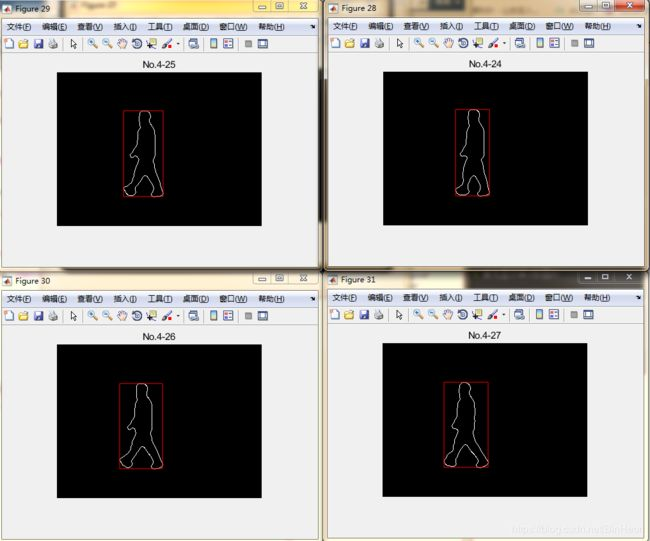展开全文• 1. 超最小二乘椭圆拟合（Hyper least squares fitting of ellipses） 上一篇博客给出了最小二乘椭圆拟合的函数（点击打开链接），超最小二乘椭圆拟合和最小二乘椭圆拟合有什么区别呢？简单来说，当所给的椭圆拟合...

1. 超最小二乘椭圆拟合（Hyper least squares fitting of ellipses）

上一篇博客给出了最小二乘椭圆拟合的函数（点击打开链接），超最小二乘椭圆拟合和最小二乘椭圆拟合有什么区别呢？简单来说，当所给的椭圆拟合数据只是占椭圆的一部分时，超最小二乘椭圆拟合的精度要高于最小二乘椭圆拟合，如下图所示。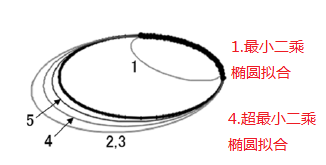详细介绍请看文献：(1) Kenichi Kanatani, Prasanna Rangarajan.Hyper least squares fitting of circles and ellipses[J], Computational Statistics and Data Analysis,2011.

2. 一般椭圆公式可以写为：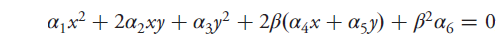3. 超最小二乘椭圆拟合函数：

%*******************************

% 函数说明：V为输入变量，是一个Nx2的矩阵，第一列和第二列分别代表x和y的值。

%                  alpha表示一般椭圆公式的系数，b表示公式中的belta。

function [alpha,b] = HLSF_5(V)
N = size(V,1);
b = max(abs(V(:)))/255;
x = V(:,1);
y = V(:,2);
Chi = [x.^2,y.^2,2*b*x,2*b*y,b^2*ones(N,1)];

X = mean(x);
Y = mean(y);
XY = mean(Chi(:,2))/2;
X2 = mean(Chi(:,1));
Y2 = mean(Chi(:,3));
W = [6*X2  X2+Y2 6*b*X 2*b*Y  b^2;...
X2+Y2 6*Y2  2*b*X  6*b*Y b^2;...
6*b*X  2*b*X 4*b^2  0     0 ;...
2*b*Y  6*b*Y 0    4*b^2  0 ;...
b^2    b^2   0     0     0];
opt.issym = true;
X = Chi'*Chi/N;
[alpha,~] = eigs(W,X,1,'lm',opt);
end

展开全文Matlab 椭圆拟合
• 描述：测量标注矩阵L每一个标注区域的一系列属性。L不同的正整数元素对应不同的区域，例如：L等于整数1的元素对应区域1；L等于整数2的元素对应区域2；以此类推。返回值STATS是一个长度为max(...

Regionprops：用途是get the properties of region，即用来度量图像区域属性的函数。

语法：STATS = regionprops(L,properties)

描述：测量标注矩阵L中每一个标注区域的一系列属性。L中不同的正整数元素对应不同的区域，例如：L中等于整数1的元素对应区域1；L中等于整数2的元素对应区域2；以此类推。返回值STATS是一个长度为max(L(:))的结构数组，结构数组的相应域定义了每一个区域相应属性下的度量。properties 可以是由逗号分割的字符串列表、饱含字符串的单元数组、单个字符串 'all' 或者 'basic'。如果 properties 等于字符串 'all'，则所有下述字串列表中的度量数据都将被计算，如果 properties 没有指定或者等于 'basic'，则属性: 'Area', 'Centroid', 和 'BoundingBox' 将被计算。下面的列表就是所有有效的属性字符串，它们大小写敏感并且可以缩写。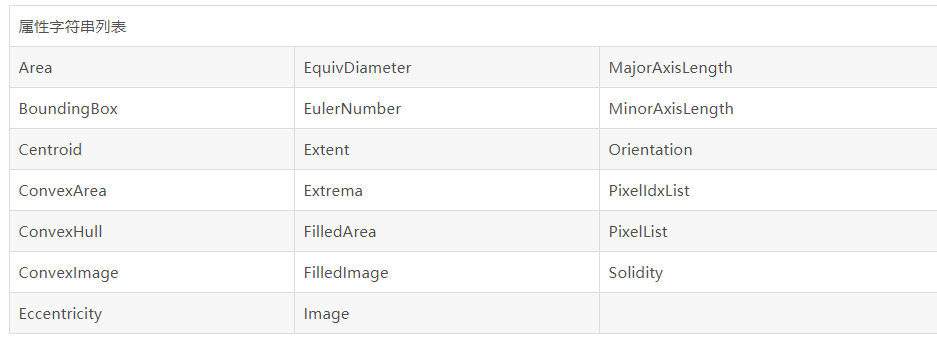属性详细定义：本部分将结合一个具体的例子说明各种字串相关属性的意义，矩阵取自在蚁蛉模式识别中做过预处理后的斑纹分割图像，如下图：

这是一幅二值图像，在应用regionprops函数之前必须将其标注，可以调用 bwlabel函数和伪彩色处理，标注后的图像如下图：

下面基于以上的材料来考察属性的含义。

'Area'：是标量，计算出在图像各个区域中像素总个数。注意：这个数值可能与由函数 bwarea 计算的值有轻微的不同。对于这样一个数值，我们可以使用它除以整个图像区域的像素个数而得到斑纹比例，可以作为模式识别的候选特征，并且这个特征是仿射不变的。

'BoundingBox'：是1行ndims(L)*2列的向量，即包含相应区域的最小矩形。BoundingBox 形式为 [ul_corner width]，这里 ul_corner 以 [x y z ...] 的坐标形式给出边界盒子的左上角、boxwidth 以 [x_width y_width ...] 形式指出边界盒子沿着每个维数方向的长度。本例的各部分区域最小矩形如下图！注意：请在这熟悉一下函数rectangle的使用方法。

'Centroid'：是1行ndims(L)列的向量，给出每个区域的质心（重心）。注意：Centroid 的第一个元素是重心水平坐标（x坐标）、第二个元素是重心垂直坐标（y坐标）。Centroid 所有其它元素则按照维顺序排列。下图采用以中心为圆心的小圆来演示质心检测的效果：

'MajorAxisLength'：是标量，与区域具有相同标准二阶中心矩的椭圆的长轴长度（像素意义下）。本属性只支持二维标注矩阵。

'MinorAxisLength'：是标量，与区域具有相同标准二阶中心矩的椭圆的短轴长度（像素意义下）。本属性只支持二维标注矩阵。

'Eccentricity'：是标量，与区域具有相同标准二阶中心矩的椭圆的离心率（可作为特征）。本属性只支持二维标注矩阵。

'Orientation'：是标量，与区域具有相同标准二阶中心矩的椭圆的长轴与x轴的交角（度）。本属性只支持二维标注矩阵。

我们可以考察离心率的变化趋势，得到对于整个区域中的各区域的似圆性如何的大致感觉，比如下图是12个区域的离心率变化情形：

由上图可以看出区域整体的似圆性并不好，实际上可以考虑使用离心率向量作为一个模式识别的特征！！

'Image'：二值图像，与某区域具有相同大小的逻辑矩阵。你可以用这个属性直接将每个子区域提取出来，然后再作相应的处理！

'FilledImage'：与上相同，唯一区别是这是个做了填充的逻辑矩阵！
本例中和上面的没有区别，只有区域有空洞时才有明显差别。

'FilledArea'：是标量，填充区域图像中的 on 像素个数。

'ConvexHull'：是p行2列的矩阵，包含某区域的最小凸多边形。此矩阵的每一行存储此多边形一个顶点的xy坐标。此属性只支持2维标注矩阵。例如：本例中的所有子区域的最小凸多边形图形如下图

看看第2个区域的大图:

'ConvexImage'：二值图像，用来画出上述的区域最小凸多边形。同时此凸包内的像素均打开，图像尺寸和此区域对应边界矩形相同。此属性只支持2维标注矩阵。注意：此处函数roipoly很有用！

'ConvexArea'：是标量，填充区域凸多边形图像中的 on 像素个数。

'EulerNumber'：是标量，几何拓扑中的一个拓扑不变量--欧拉数，等于图像中目标个数减去这些目标中空洞的个数。此属性只支持2维标注矩阵。本例中的欧拉数均为1。

'Extrema'：8行2列矩阵，八方向区域极值点。矩阵每行存储这些点的xy坐标，向量格式为 [top-left top-right right-top right-bottom bottom-right bottom-left left-bottom left-top]。此属性只支持2维标注矩阵。

'EquivDiameter'：是标量，等价直径：与区域具有相同面积的圆的直径。计算公式为：sqrt(4*Area/pi)。. 此属性只支持2维标注矩阵。

'Solidity'：是标量，同时在区域和其最小凸多边形中的像素比例。计算公式为：Area/ConvexArea，这也是个仿射特征，实际上反映出区域的固靠性程度。此属性只支持2维标注矩阵。

'Extent'：是标量，同时在区域和其最小边界矩形中的像素比例。计算公式为：Area除以边界矩形面积，这也是个仿射特征，实际上反映出区域的扩展范围程度。此属性只支持2维标注矩阵。不再给出计算结果！！

'PixelIdxList'：p元向量，存储区域像素的索引下标。

'PixelList'：p行ndims(L)列矩阵，存储上述索引对应的像素坐标。

支持类：输入的标注矩阵L可以有任意的数值类型。

提醒

使用逗号分割列表语法：当你基于regionprops函数的输出作算法设计时，使用逗号分割列表语法就凸显出其非常的价值。例如：对于一个存储标量的属性，可以利用此语法创建一个包含图像中不同区域内此属性值的向量。例如以下两句是等价的：

stats(1).Area, stats(2).Area, ..., stats(end).Area
stats.Area
因此，可以使用下面的方法创建相应的向量：

regionprops(L,'Area'); allArea = [stats.Area];
allArea 就是一个与结构数组 stats 具备相同长度的向量。

基于特定原则的区域选择：当你要基于特定准则条件选择某个区域时，将函数 ismember 和 regionprops 联合使用是很有用处的。例如：创建一个只包含面积大于80的二值图像，用以下命令

idx = find([stats.Area] > 80); BW2 = ismember(L,idx);
计算性能考虑：大多数的属性测量计算时间都非常地少，除了那些非常依赖于图像L中区域个数和像素个数的属性。例如：

'ConvexHull' 'ConvexImage' 'ConvexArea' 'FilledImage'
另外建议一次性计算所有属性值，因为分开计算和一起计算时间相差无几！

使用二值图像工作：在调用regionprops之前必须将二值图像转变为标注矩阵。两个函数可以做到：

L = bwlabel(BW); L = double(BW);
注意：虽然这两个函数从同一二值图像产生不同的标注矩阵，但是它们是等效的！例如：给出如下的二值矩阵BW，

1 1 0 0 0 0 1 1 0 0 0 0 0 0 0 0 0 0 0 0 0 0 1 1 0 0 0 0 1 1
bwlabel 创建一个包含两个分别由整数1和2标注的连续区域标注矩阵

mylabel = bwlabel(BW) mylabel = 1 1 0 0 0 0 1 1 0 0 0 0 0 0 0 0 0 0 0 0 0 0 2 2 0 0 0 0 2 2
double 创建一个包含一个由整数1标注的不连续区域标注矩阵。

mylabel2 = double(BW) mylabel2 = 1 1 0 0 0 0 1 1 0 0 0 0 0 0 0 0 0 0 0 0 0 0 1 1 0 0 0 0 1 1
regionprops 并不负责自动转换二值图像数据类型，而是由你自己决定使用何种数据转换方法来存储自己想要的数据。

regionprops函数的扩展思路：在regionprops函数的基础上，你可以使用它提供的基本数据来扩展它的功能，将区域的曲率数据和骨架数据作为它的另外属性值来开发，从而希望它能用来做更细致的特征提取。
———————————————

获取图像中的连通区域，可以使用以下代码：

src_img_name = '1.jpg';

% get binary image
gray_img = rgb2gray(img);
T = graythresh(gray_img);
bw_img = im2bw(gray_img, T);

% find the largest connected region
img_reg = regionprops(bw_img,  'area', 'boundingbox');
areas = [img_reg.Area];
rects = cat(1,  img_reg.BoundingBox);

显示所有连通区域

% show all the largest connected region
figure(1),
imshow(bw_img);
for i = 1:size(rects, 1)
rectangle('position', rects(i, :), 'EdgeColor', 'r');
end

显示最大连通区域：

[~, max_id] = max(areas);
max_rect = rects(max_id, :);

% show the largest connected region
figure(2),
imshow(bw_img);
rectangle('position', max_rect, 'EdgeColor', 'r');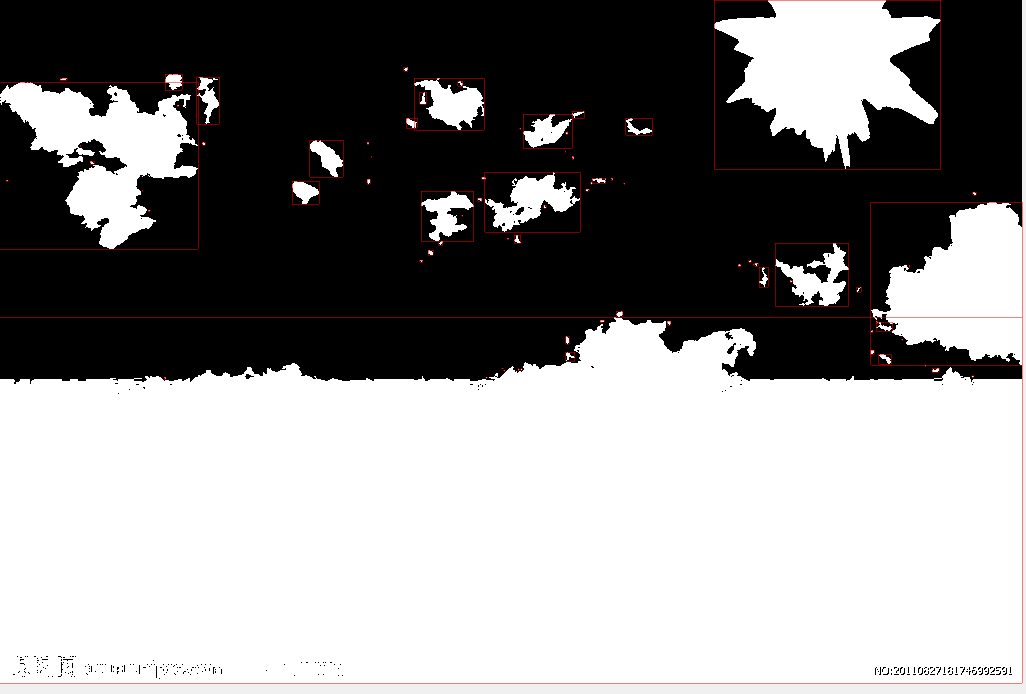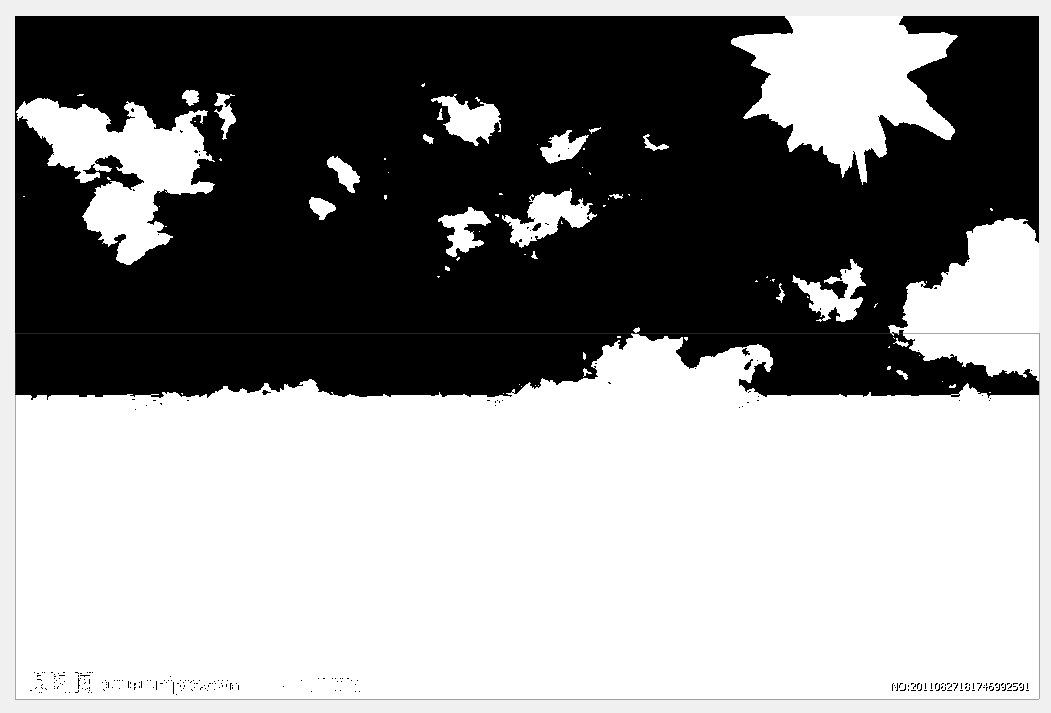展开全文• 直接最小二乘法拟合椭圆

万次阅读 多人点赞 2018-09-18 21:40:25
这里写自定义目录标题直接最小二乘法拟合椭圆椭圆方程优化目标拉格朗日函数根据特征值求解参数 直接最小二乘法拟合椭圆 利用最小二乘算法构造方程，使用拉格朗日乘子进行求解 椭圆方程 ax2+bxy+cy2+dx+ey=1 ax^...

直接最小二乘法拟合椭圆

利用最小二乘算法构造方程，使用拉格朗日乘子进行求解

椭圆方程

A x 2 + B x y + C y 2 + D x + E y + F = 0 Ax^2+Bxy+Cy^2+Dx+Ey+F=0

优化目标

W = [ A , B , C , D , E , F ] ⊤ W=\left[A,B,C,D,E,F\right]^\top X = [ x 2 , x y , y 2 , x , y , 1 ] ⊤ X=\left[x^2,xy,y^2,x,y,1\right]^\top ，则优化目标为
min ⁡ ∥ W ⊤ X ∥ 2 = W ⊤ X X ⊤ W s . t . W ⊤ H W &gt; 0 \min\left\|{W^\top X }\right\|^2 =W^\top X X^\top W\\ s.t. \quad W^\top H W&gt;0
其中 H = [ 0 0 2 0 0 0 0 − 1 0 0 0 0 2 0 0 0 0 0 0 0 0 0 0 0 0 0 0 0 0 0 0 0 0 0 0 0 ] H = \begin{bmatrix} 0 &amp; 0 &amp; 2 &amp; 0 &amp; 0 &amp; 0 \\ 0 &amp; -1 &amp; 0 &amp; 0 &amp; 0 &amp; 0 \\ 2 &amp; 0 &amp; 0 &amp; 0 &amp; 0 &amp; 0 \\ 0 &amp; 0 &amp; 0 &amp; 0 &amp; 0 &amp; 0 \\ 0 &amp; 0 &amp; 0 &amp; 0 &amp; 0 &amp; 0 \\ 0 &amp; 0 &amp; 0 &amp; 0 &amp; 0 &amp; 0 \\ \end{bmatrix}
W ⊤ H W &gt; 0 \quad W^\top H W&gt;0 是椭圆参数约束 4 A C − B 2 &gt; 0 4AC-B^2&gt;0

由于 ∥ W ⊤ X ∥ 2 = 0 \left\|{W^\top X }\right\|^2=0 时， W W 可以有一个缩放因子，即所有 W ′ = α W W^\prime = \alpha W 也同样满足条件，因此我们让 W ⊤ H W = 1 \quad W^\top H W=1

于是优化目标变为：
min ⁡ ∥ W ⊤ X ∥ 2 = W ⊤ X X ⊤ W s . t . W ⊤ H W = 1 \min\left\|{W^\top X }\right\|^2 =W^\top X X^\top W\\ s.t. \quad W^\top H W=1

拉格朗日函数

构造拉格朗日函数
L ( W , λ ) = W ⊤ X X ⊤ W − λ ( W ⊤ H W − 1 ) L\left(W,\lambda\right)=W^\top X X^\top W-\lambda \left( W^\top H W-1\right)
对其求导得零：
∂ L ∂ W = 0 \frac{{\partial L}}{{\partial W}} = 0

X X ⊤ W − λ H W = 0 ⇒ X X ⊤ W = λ H W XX^\top W-\lambda HW = 0 \Rightarrow XX^\top W=\lambda HW
S = X X ⊤ S=XX^\top ，则 S W = λ H W SW=\lambda HW ，通过求解广义特征向量可以得到6个可能的备选 W W 。然后需要用到 W ⊤ H W = 1 W^\top H W=1 这个条件来筛选合格的 W W 。由于 u W uW 也满足 S u W = λ H u W SuW=\lambda HuW ，要使 u W ⊤ H u W = 1 uW^\top HuW=1 u = 1 W ⊤ H W = λ W ⊤ S W u=\sqrt{\frac{1}{W^\top HW}}=\sqrt{\frac{\lambda}{W^\top SW}} ，由于 S S 是正定矩阵，所以 λ &gt; 0 {\lambda&gt;0} 。因此在特征值大于0的特征向量里面选出那些实特征向量即可满足要求，并计算对应的缩放系数 u u 。详见论文"Direct least square fitting of ellipses"。

如果不求广义特征向量，由于 S S 为正定矩阵，也可以将 S S 的逆乘到左右两边，得
S − 1 H W = 1 λ W {S^{ - 1}}HW = \frac{1}{\lambda }W
这就转换为求解特征向量的问题。

更早的一种直接拟合法

优化目标

min ⁡ ∥ W ⊤ X ∥ 2 = W ⊤ X X ⊤ W s . t . W ⊤ W = 1 \min\left\|{W^\top X }\right\|^2 =W^\top X X^\top W\\ s.t. \quad W^\top W=1
这里 W ⊤ W = 1 W^\top W=1 是为了避免 W = 0 W=0 的情形，但也可以看出，这种方法不能保证结果一定是椭圆，可能是其他二次曲线。

拉格朗日函数

构造拉格朗日函数
L ( W ) = W ⊤ X X ⊤ W − λ ( W ⊤ W − 1 ) L\left(W\right)=W^\top X X^\top W-\lambda \left( W^\top W-1\right)
对其求导得零：
∂ L ∂ W = 0 \frac{{\partial L}}{{\partial W}} = 0

X X ⊤ W − λ W = 0 ⇒ X X ⊤ W = λ W ⇒ S W = λ W XX^\top W-\lambda W = 0 \Rightarrow XX^\top W=\lambda W \Rightarrow S W=\lambda W
然后求解 S S 的特征向量即可，但由于有6个特征向量，因此需要筛选符合要求的特征向量

筛选符合要求的特征向量

假设得到特征值和特征向量对 { λ i , v i } \left\{ {{\lambda _i},{v_i}} \right\}

此外，对于椭圆方程
a x 2 + 2 h x y + b y 2 + 2 g x + 2 f y + c = 0 ax^2+2hxy+by^2+2gx+2fy+c=0
判别式
Δ = ∣ a h g h b f g f c ∣ = a b c + 2 f g h − a f 2 − b g 2 − c h 2 \Delta=\begin{vmatrix} a&amp;h&amp;g \\ h&amp;b&amp;f \\ g&amp;f&amp;c \\ \end{vmatrix}=abc+2fgh-af^2-bg^2-ch^2
Δ ≠ 0 \Delta\ne0 ，且 a b − h 2 &gt; 0 ab-h^2&gt;0 时为椭圆

条件一 Δ ≠ 0 \Delta\ne0

条件二 a b − h 2 &gt; 0 ab-h^2&gt;0 v i ⊤ H v i &gt; 0 v_i^\top Hv_i&gt;0

对于实椭圆， Δ a + b &lt; 0 \frac{\Delta}{a+b}&lt;0

条件三 Δ a + b &lt; 0 \frac{\Delta}{a+b}&lt;0

符合上面三个条件的特征向量可以作为椭圆方程的参数

还有另一种筛选方法，但不如上述方法严格，由于 W ⊤ X X ⊤ W W^\top XX^\top W 为二次误差，那么使二次误差最小的特征向量应该是椭圆的参数向量。由于
X X ⊤ W = λ W ⇒ W ⊤ X X ⊤ W = λ W ⊤ W XX^\top W=\lambda W \Rightarrow W^\top XX^\top W = \lambda W^\top W
W ⊤ H W &gt; 0 W^\top H W&gt;0 ，所以最小的特征值 λ i \lambda_i 对应的特征向量即为椭圆参数向量。

根据椭圆一般方程求解椭圆参数

椭圆方程：
A x 2 + B x y + C y 2 + D x + E y + F = 0 Ax^2+Bxy+Cy^2+Dx+Ey+F=0
几何中心：
X c = B E − 2 C D 4 A C − B 2 Y c = B D − 2 A E 4 A C − B 2 \begin{aligned} X_c&amp;=\frac{BE-2CD}{4AC-B^2}\\ Y_c&amp;=\frac{BD-2AE}{4AC-B^2} \end{aligned}
长半轴短半轴：
A 2 = 2 ( A X c 2 + C Y c 2 + B X c Y c − F ) A + C + ( A − C ) 2 + B 2 B 2 = 2 ( A X c 2 + C Y c 2 + B X c Y c − F ) A + C − ( A − C ) 2 + B 2 \begin{aligned} A^2 = \frac{2\left(AX_c^2+CY_c^2+BX_cY_c-F\right)}{A+C+\sqrt{\left(A-C\right)^2+B^2}}\\ B^2 = \frac{2\left(AX_c^2+CY_c^2+BX_cY_c-F\right)}{A+C-\sqrt{\left(A-C\right)^2+B^2}} \end{aligned}
长轴倾角：
θ = 1 2 arctan ⁡ B A − C \theta=\frac{1}{2}\arctan\frac{B}{A-C}
上述方法有可能求出来的是短轴的倾角，因为公式并没有区分两个轴的长短，更稳妥的算法如下方python代码所示：

#A*x.^2 + B*x.*y + C*y.^2 + D*x + E*y + F
def solve_ellipse(A,B,C,D,E,F):

Xc = (B*E-2*C*D)/(4*A*C-B**2)
Yc = (B*D-2*A*E)/(4*A*C-B**2)

FA1 = 2*(A*Xc**2+C*Yc**2+B*Xc*Yc-F)
FA2 = np.sqrt((A-C)**2+B**2)

MA = np.sqrt(FA1/(A+C+FA2)) #长轴
SMA= np.sqrt(FA1/(A+C-FA2)) if A+C-FA2!=0 else 0#半长轴

if B==0 and F*A<F*C:
Theta = 0
elif B==0 and F*A>=F*C:
Theta = 90
elif B!=0 and F*A<F*C:
alpha = np.arctan((A-C)/B)*180/np.pi
Theta = 0.5*(-90-alpha) if alpha<0 else 0.5*(90-alpha)
else:
alpha = np.arctan((A-C)/B)*180/np.pi
Theta = 90+0.5*(-90-alpha) if alpha<0 else 90+0.5*(90-alpha)

if MA<SMA:
MA,SMA = SMA,MA

return [Xc,Yc,MA,SMA,Theta]

Matlab代码

生成椭圆散点数据

%% parameters of the true ellipse
t = 0:1:120;
xs = 6*cosd(t);
ys = 21*sind(t);
noise = randn(2,length(xs))*0.5;
xs = xs+noise(1,:);
ys = ys+noise(2,:);
M_z = rotz(10);
M_z = M_z(1:2,1:2);
new_X = M_z*[xs; ys];
xs = new_X(1,:)+5;
ys = new_X(2,:)+4;
figure(1)
clf
scatter(xs,ys,[],'.');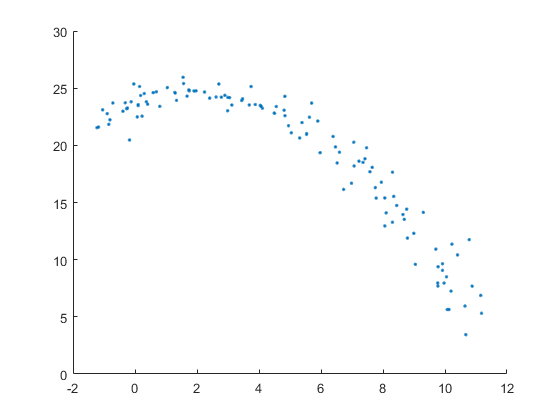拟合椭圆

X = [xs.^2;
xs.*ys;
ys.^2;
xs;
ys;
ones(1,length(xs))];
H = zeros(6);
H(1,3)=2;
H(3,1)=2;
H(2,2)=-1;
S = X*X';

算法1：

[V,L] = eig(S,H)
L = diag(L);

绘制椭圆

for i=1:6
if L(i)<=0
continue;
end

W = V(:,i);

if W'*H*W<0
continue
end

W = sqrt(1/(W'*H*W))*W

A = W(1); B = W(2); C = W(3); D = W(4); E = W(5); F = W(6);
funs = @(x,y) A*x.^2 + B*x.*y + C*y.^2 + D*x + E*y + F;
figure;
hold on;
scatter(xs,ys,[],'.');
fimplicit(funs)

Xc = (B*E-2*C*D)/(4*A*C-B^2)
Yc = (B*D-2*A*E)/(4*A*C-B^2)

MA = sqrt(2*(A*Xc^2+C*Yc^2+B*Xc*Yc-F)/(A+C+sqrt((A-C)^2+B^2)))
SMA= sqrt(2*(A*Xc^2+C*Yc^2+B*Xc*Yc-F)/(A+C-sqrt((A-C)^2+B^2)))
end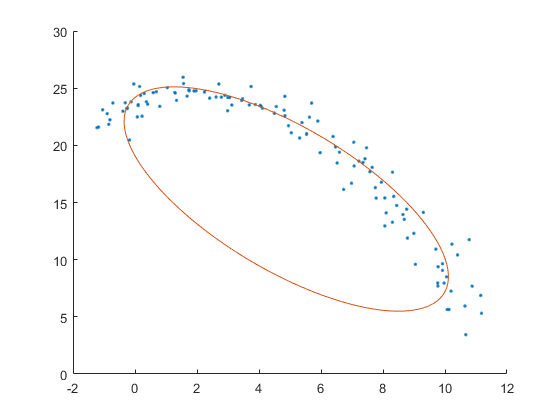Xc = 4.8793
Yc = 15.3049
MA = 3.5116
SMA = 10.5489

算法2：

[V,L] = eig(S)
E = zeros(1,6)
for i=1:size(V,2)
E(i) = V(:,i)'*S*V(:,i);
end
E
[~,I] = min(E);
W = V(:,I)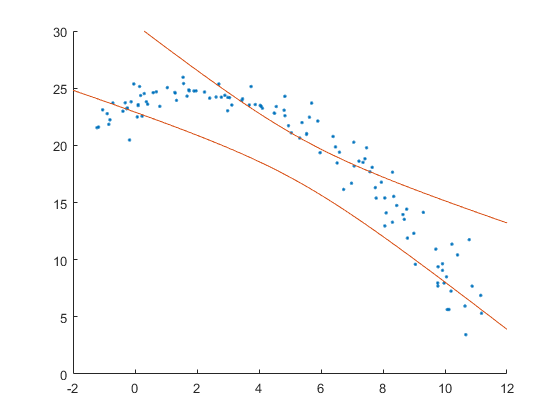上面是二次误差最小的二次曲线，下面是二次误差第二小的二次曲线，一个是双曲线，一个是抛物线，明显不符合要求。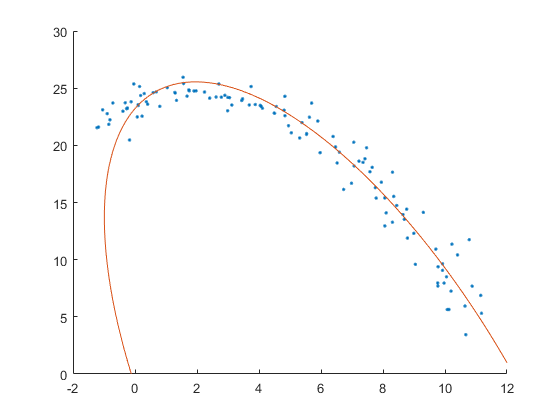因为散点只取了一小段，所以两个算法精度都很差，若是比较完整的数据，则两个算法结果差不多。

参考链接

展开全文椭圆 最小二乘 拉格朗日
• 摘 要： 提出一种基于最小外包矩形的快速椭圆拟合方法，该方法利用最小二乘法获得目标的最小外包矩形框，再求取外包矩形框的内切椭圆，该椭圆能有效反映目标的大部分运动信息。本文对该方法进行了目标拟合的有效性和...
• Matlab最小面积包围四边形

千次阅读 2017-04-12 21:23:01matlab 包围盒
• Python 中文教程15——OpenCV 的轮廓参考博客：一、初识轮廓目标• 理解什么是轮廓• 学习找轮廓，绘制轮廓等• 函数： cv2.findContours()， cv2.drawContours() 1、什么是轮廓 轮廓可以简单认为成将连续的点...opencv中的轮廓 数字图像处理
• 新手，不懂什么叫精华帖，哪里可以看到这些，这样是不是清楚一些呢function Z=dingyayujin7003(n,f)fa=300;...%%%轴承径db=5.4/1000;%%%%滚球直径den=3.2e3; %%%%%%%%%%%%%%%%%%%%钢球密度v1=0.3;%%%%%%%%%%%...
• 标量 Orientation 与区域具有相同标准二阶中心矩的椭圆的长轴与x轴的交角（度） 标量 ConvexArea 填充区域凸多边形图像的像素个数 标量 ConvexHull 获取包含某区域的最小凸多边形 p*2 ConvexImage 用来出上述的...
• 1、 matlab函数bwareaopen──删除小面积对象格式：BW2 = bwareaopen(BW,P,conn)作用：删除二值图像BW面积小于P的对象，默认情况下使用8邻域。算法：(1)Determine the connected components.L = bwlabel(BW, conn)...
• 通俗的说，这个函数的作用是用来找这个二值图像的连通区域的，对于不同的符合条件的连通区域（4连通，8连通）分别用不同的标号加以区别，结果保存L这个矩阵里，而num里保存的是输入图像连通区域的总数。...Matlab 形状识别 图像处理 连通域
• 此文章处，将讨论特征以及怎样利用视觉工具箱从图像提取特征。借助于上一篇博客的图像处理技术，我们将要讨论几种特征类型:区域、线和兴趣点。 区域特征 图像分割是把一幅图像划分成具有应用意义的若干区域的...计算机视觉 机器学习 matlab c++ 图像识别
• 基于matlab的图像形状与分类摘 要数字图像处理是一门新兴技术，随着计算机硬件的发展，数字图像的实时处理已经成为可能，由于数字图像处理的各种算法的出现，使得其处理速度越来越快，能更好的为人们服务。...计算机视觉 机器学习 人工智能 算法 编程语言
• 摘 要 目前，随着人们生活水平的提高，各国人民汽车保有量也越来越多，伴随而来的是交通事故也不断增多。研究表明，疲劳驾驶是造成交通事故日益严重的重要原因。开展驾驶员疲劳检测和预警的研究工作，有着十分重要...算法 定位 控制器 dbcp webgl
• OpenCV （Open Source Computer Vision Library）的相关简介，OpenCv 可以运行多平台之上，轻量级而且高效，由一系列 C 函数和少量 C++类构成，提供了 Python、Ruby、MATLAB 等语言的接口，所以学习的时候，要...python 计算机视觉 机器学习 人工智能 opencv
• matlab程序

千次阅读 2008-12-10 19:36:00matlab image plot spring disk
• 这也算是解析几何的一大奇技淫巧~一、定义仿射变换，又称仿射映射，是指几何，一个向量空间进行一次线性变换并接上一个平移，变换为另一个向量空间。以下称变换 ， 为圆锥曲线标准变换。经过标准变换后，椭圆 ...
• OpenCV-Python (官方)中文教程(部分一)

千次阅读 多人点赞 2020-01-13 13:54:06
官网链接(英文版):... 第一章.OpenCV简介 了解如何计算机上设置OpenCV-Python！ 1.OpenCV-Python教程简介 1.1 OpenCV ...OpenCV是由Gary Bradsky于1999年Intel公司创建的，第一次发布是2000年。瓦...OpenCV 图像处理 Python
• OpenCV中的drawContours使用

万次阅读 2016-06-01 09:37:20
基于内容的图像分析的重点是提取出图像具有代表性的特征，而线条、轮廓、块往往是最能体现特征的几个元素，这篇文章就针对于这几个重要的图像特征，研究它们OpenCV的用法，以及做一些简单的基础应用。...opencv
• 前言：opencv已经更新到3.1.0的版本，此文列出其2.0版本时代的库函数，多数函数与3.0以上版本有着相似之处，比如很多2.0版本函数名去掉前缀cv就是3.0版本的函数名cvWaitKey变成Waitkey(),cvNamedWindow变成...函数 图像处理 索引 matlab opencv
• 函数的代码是部分代码，详细代码最后 1 cv2.boundingRect 作用：矩形边框（boundingRect），用于计算图像一系列点的外部矩形边界。 cv2.boundingRect(array) -> retval 参数： array - 灰度图像...
• 在上一篇文章有提到sobel边缘检测，并重写了soble的C++代码让其与matlab中算法效果一致，而soble边缘检测是基于单一阈值的，我们不能兼顾到低阈值的丰富边缘和高阈值时的边缘缺失这两个问题。而canny算子则很好的...opencv检测直线 opencv提取轮廓 轮廓描述 霍夫变换
• 特别之处在于能快速检测图像的线段，同时使用了错误控制的方法，使得检测结果比较准确。最后，LSD结果输出是线段的坐标与属性，比如起点，终点，线段的粗细。  首先，我们需要回顾一下，为什么需要检测图像...
• ==================================前言：opencv已经更新到3.1.0的版本，此文列出其2.0版本时代的库函数，多数函数与3.0以上版本有着相似之处，比如很多2.0版本函数名去掉前缀cv就是3.0版本的函数名cvWaitKey变成...
• reconstruct)#拟合椭圆 cv2.ellipse(img, ellipse, (0, 0, 255), 2) df = pd.DataFrame(np.random.rand(10,4), columns = [u'外接矩形',u'最小外接矩阵',u'外接圆',u'椭圆']) fig = df.plot(figsize = (6,6)) #创建...opencv 图像识别 计算机视觉 边缘检测
• 一、Canny检测轮廓在上一篇文章有提到sobel边缘检测，并重写了soble的C++代码让其与matlab中算法效果一致，而soble边缘检测是基于单一阈值的，我们不能兼顾到低阈值的丰富边缘和高阈值时的边缘缺失这两个问题。...c语言图像轮廓坐标matlab如何在最小外接矩阵中画椭圆matlab 订阅